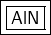## What is the formula for the compound formed by Al3+ and N3- ? ​

Question

What is the formula for the compound formed by Al3+ and N3- ?

in progress 0
6 months 2021-07-11T13:20:50+00:00 1 Answers 1 views 0Explanation:

The goal when forming compounds is to achieve a net charge of 0.

We are given aluminum with a charge of 3+  and nitrogen with a charge of 3-.

These charges are equal and opposite, so one of each will equal 0.

• 3+ (Al) + 3- (N) = 0

Therefore, we do not need any subscripts. List the metal’s symbol (aluminum) then the nonmetal.

• AlN

Th formula for the compound, which is aluminum nitride, is AlN.